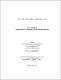## Covering points with axis parallel lines2015
##### Authors
Jahan, Kawsar
University of Lethbridge. Faculty of Arts and Science
##### Publisher
Lethbridge, Alta : University of Lethbridge, Dept. of Mathematics and Computer Science
##### Abstract
In this thesis, we study the problem of covering points with axis parallel lines. We named it as the point cover problem. Given a set of the n points in d dimensional space, the goal is to cover the points with minimum number of axis parallel lines. We use the iterative rounding approach which gives an integral solution to the problem. We also propose a combinatorial algorithm by using the branch and bound technique which gives an optimal integral solution to the point cover problem. Later we implemented another approximation algorithm based on primal-dual and iterative rounding approaches. Finally we show the comparative analysis between the two iterative rounding algorithms.
##### Keywords
3 dimensional space , axis parallel lines , branch and bound , covering points